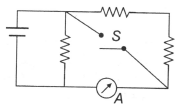In the circuit shown, the reading of the ammeter is doubled after the switch is closed. Each resistor has a resistance equal to 1  Ω and the ideal cell has an emf equal to 10 V. Then the ammeter has a resistance equal to

# In the circuit shown, the reading of the ammeter is doubled after the switch is closed. Each resistor has a resistance equal to $1\text{\hspace{0.17em}\hspace{0.17em}}\Omega$ and the ideal cell has an emf equal to 10 V. Then the ammeter has a resistance equal to1. A

$2\text{\hspace{0.17em}\hspace{0.17em}}\Omega$

2. B

$1\text{\hspace{0.17em}\hspace{0.17em}}\Omega$

3. C

$2.5\text{\hspace{0.17em}\hspace{0.17em}}\Omega$

4. D

none of these

Register to Get Free Mock Test and Study Material

+91

Verify OTP Code (required)

### Solution:

$\begin{array}{l}{\mathrm{I}}_{1}=\frac{10}{2+{\mathrm{R}}_{\mathrm{A}}},\text{\hspace{0.17em}\hspace{0.17em}}{\mathrm{I}}_{2}=\frac{10}{{\mathrm{R}}_{\mathrm{A}}}\text{\hspace{0.17em}\hspace{0.17em}}\mathrm{and}\text{\hspace{0.17em}\hspace{0.17em}}{\mathrm{I}}_{2}=2{\mathrm{I}}_{1}\\ \mathrm{Solving},\text{\hspace{0.17em}\hspace{0.17em}}\mathrm{we}\text{\hspace{0.17em}\hspace{0.17em}}\mathrm{get}\text{\hspace{0.17em}\hspace{0.17em}}{\mathrm{R}}_{\mathrm{A}}=2\text{\hspace{0.17em}}\mathrm{\Omega }\end{array}$Register to Get Free Mock Test and Study Material

+91

Verify OTP Code (required)